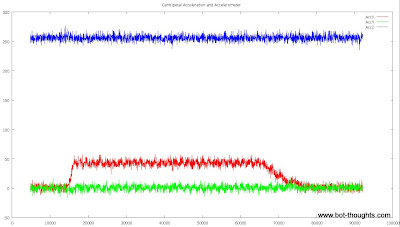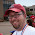Tuesday, April 24, 2012

AHRS, IMU and AccelerationWorld and Car axes
In working on my 2012 AVC entry, Data Bus, I've been evaluating the use of a 3D IMU (Inertial Measurement Unit) and AHRS (attitude heading reference system) to provide an accurate heading estimate.

An IMU is comprised of some combination of gyros, accelerometers, and magnetometers and can provide enough sensor data to estimate attitude and heading in 2D or 3D.

These sensors plus onboard processing to determine attitude and heading, is called an Attitude and Heading Reference System (AHRS).

The AHRS fuses sensor data to determine which way is North and which way is "Down" and then it computes heading and vehicle attitude.

That is, provided it doesn't change velocity or direction....

Let's back up a second and talk more about how an AHRS works.

Gyro sensors measure rotation around an axis. A 3-axis gyro measures rotation around the x, y, and z axis, respectively. The problem with gyros is that they tend to drift. So a 3D, absolute reference is needed to correct for drift. This is where the "Down" and North references come into play.

Magnetometers provide the North reference and accelerometers, measuring the Earth's gravitational acceleration vector, provide the "Down" reference. Provided the AHRS is stationary. The problem is acceleratometers. They measure acceleration due to gravity as well as the vehicle's acceleration.

Try this experiment. Hang a small weight on a string from your rear view mirror, then have someone watch it as you drive straight at a constant speed, then as you accelerate, and finally as you drive around a corner.

Does the string always point down? No.

As you go around a corner, it points down and slightly to the outside of the corner.  When you accelerate it points down and slightly rearward. That string is what happens to the "down" reference vector.

How big of a deal is it? Time for math, Octave (Matlab), and Gnuplot fun...

Centripetal Acceleration

Centripetal acceleration is perpendicular to the tangential direction of travel of a vehicle going around a corner. How big of a deal is it? Setting up a test is easy...Breadboard Arduino AHRS with minIMU-9 ready for testing
On a breadboard, I've prototyped an Arduino AHRS using the Pololu minIMU-9 sensor and example code from Pololu. The setup logs raw accelerometer data to a microSD card.

I placed the rig on my vintage turntable platter with the X-axis of the sensor oriented radially. Then, I powered up the AHRS and let it collect data while stationary for about 10 seconds. Next, I powered up the turntable and it spun up to approximately 33-1/3 RPM. After 50 seconds or so, I stopped the turntable. Then the platter slows down for 10-20 seconds.Turntable test: x, y, and z accelerometer values
Lo and behold, the plot shows the expected behavior (don't you love it when your lab experiments actually work?).

The Y and Z axes (blue and green) show no major disturbance as they were not acted on by much acceleration.

The X axis (red), shows a quick ramp up from a zero point to a higher value (as the turntable increased rotational speed), then it holds for 50 seconds, then gradually decreases to zero while the platter slows.

Accelerometer Reference Error

To find out just how big of a deal this X-axis acceleration is, let's see how the attitude estimate is affected by plotting the X- and Y-axis components of the vector versus time.Turntable test: accelerometer x-z and y-z angles
Not surprisingly, all the error is in the X component (the red plot) and there's about 10 degrees of error when this centripetal acceleration is present.

Ok, how much centripetal acceleration was our test rig seeing? The sensors show about 250 in the z axis. That's 1g.  The x-axis was seeing a value of 50.  That works out to about 0.2g.  Let's calculate and see what happens.

Calculating Centripetal Acceleration

Centripetal acceleration is given by
and

Where v is the tangential velocity and r is radius, in our case about 6" (0.1524m) and T is the period of rotation. At an angular velocity of 33-1/3 rev/min, T=0.03min/rev or 1.8s/rev, so v=0.532m/s, giving  a=1.857m/s2 or 0.19g, which matches the sensor reading above.

Let's say Data Bus takes a turn at a leisurely 10mph (4.47m/s) and the turn radius is a rather wide 4m. That works out to about 5m/s2 or 0.51g. Presumably the X-Z vector will show even more error.

Tilt Compass Error

Even though 10+ degrees of error in the "down" reference vector sounds like a lot, how much does it impact heading estimate?  For that matter, how are reference vector error and heading estimate error related?

First, let's calculate a simple tilt-compensated heading using Octave, for a particular scenario: an imaginary vehicle on perfectly flat ground with an actual heading of 45 degrees experiencing 0.2g centripetal acceleration. The calculation simply uses the accelerometers for a "down" reference.

The measured acceleration vector (x, y, z) in g is:

a = [ 0; 0.20; -1 ]

The magnetometer vector (normalized to magnitude of 1) is:

m = [ cos(radians(45)); sin(radians(45)); 0]
m = [ 0.7071; 0.7071; 0 ]

Note that radians() is a function you have to write toconvert degrees to radians: r = 45 * pi() / 180

From Freescale App Note 4248:

roll  = atan2( G(y), G(z) );
pitch = atan2( -G(x), G(y)*sin(roll) + G(z)*cos(roll) );
yaw   = atan2( B(z)*sin(roll)-B(y)*cos(roll), B(x)*cos(pitch)
+ B(y)*sin(pitch)*sin(roll)+B(z)*sin(pitch)*cos(roll));


(Incidentally, I've defined constants x=1, y=2, z=3 for convenience to act as indices for these 3d matrices, so G(y) is simply the 2nd element of the G matrix). Makes the equation more self-explanatory.

Converted to degrees we get roll, pitch, yaw values:

   168.69007
0.00000
44.43824

So the heading is off by about 0.6 degrees. It so happens that, at 45 degrees actual heading, the error is at its maximum. At an actual heading of 0 or 90, the error is zero. Here's a plot of heading error versus actual heading. You can see that it peaks at 45 degrees:Tilt heading error for h=0-90 deg, 0.2g centripetal acceleration
To get the big picture, we'll plot maximum error (actual heading, 45 degrees) versus centripetal acceleration (ranging from 0 to 3g).Heading error vs. Centripetal Acceleration
As you can see, error rapidly grows. Remember this is for a simple attitude estimate based directly and instantly on the accelerometer readings.

Here's the Octave script that produces the data for this blog post:

AccelMag.m

Linear Acceleration

After writing the script to compute error vs. centripetal acceleration, it's easy to tweak it to compute error vs. linear acceleration, too. Instead of varying Ay, vary Ax from 0 to 3g. The Octave script above incorporates that calculation too. Here's the result.Heading error vs. Linear Acceleration
Conclusion

It seems pretty clear to me, now, that if you're going to use a tilt-compensated 3d compass for navigation, accounting for centripetal and linear acceleration is a good idea. Mind you, the calculations above were based on unfiltered accelerometer data with no fancy attitude estimation algorithms using gyros and such.

An AHRS filters and fuses data and this filtering will delay the skewing effects of linear and/or centripetal acceleration. Simultaneously, response to higher frequency orientation changes will be curtailed. The AHRS will be less responsive.

A compromise must be chosen. As an example, MatrixPilot includes an AHRS algorithm that is essentially a complimentary filter with a time constant chosen to address the acceleration issue.

Further analysis and testing is needed to determine the best compromise between response and acceleration-induced errors.

For now, this is a moot point as the above and other analysis has led me to abandon the potential complexity of a 3d solution in favor of a simpler 2d solution.

1.Hi again.
Excellent post!

I am not sure I get what you are saying with the heading error graph though. I believe the error is the same in heading to wherever the robot is facing. Did you kept the acceleration vector the same? Because with different heading the centripetal force (being orthogonal to the velocity) should be different.

Hope I make sense!

Thanks!

2.@psychoul: I think I get what you're saying. In the vehicle frame of reference, the 'down' reference vector is always pointed down-ish, and slightly towards the center of the turn (which is in a direction perpendicular to the vehicle's axis). The robot thinks that it is tilted by some small amount and computes the heading from the magnetometer accordingly. At a heading of 0 (where the magnetic vector is maximum along the vehicle X axis and minimum along the vehicle Y axis, the accelerometer error is all along the Y axis. At a heading of 90 degrees, the magnetic vector is maximum along the Y axis and minimum along the X and the accelerometer is along the X axis. But at 45 degrees, there's equal magnetic vector and equal accelerometer error along both axes.

3.Hello!
I am sam.
Nice to meet you, first of all sorry for my bad English.